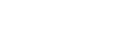## Questions and Answers per Month

0

(click on this box to dismiss)Q&A for Ubuntu users and developers

``````select [TimeOfDay],
sum(case when dow = 1 then 1 else 0 end) Sunday,
sum(case when dow = 2 then 1 else 0 end) Monday,
sum(case when dow = 3 then 1 else 0 end) Tuesday,
sum(case when dow = 4 then 1 else 0 end) Wednesday,
sum(case when dow = 5 then 1 else 0 end) Thursday,
sum(case when dow = 6 then 1 else 0 end) Friday,
sum(case when dow = 7 then 1 else 0 end) Saturday
from
(
select
cast(
right('0' + convert(varchar(2), DATEPART(HOUR, CreationDate)), 2) +
':' +
right('0' + convert(varchar, DATEPART(MINUTE, CreationDate) / 15 * 15), 2)
as datetime
) as [TimeOfDay],
convert(int, DATEPART(WEEKDAY, CreationDate)) as dow
from Posts p
where PostTypeId = 2
) as X
group by [TimeOfDay]
order by [TimeOfDay] asc``````

### Enter Parameters

Options:
-Hold tight while we fetch your results
:records returned in :time ms:cached